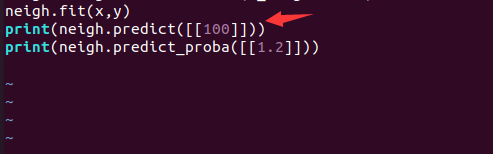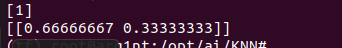# 0x00 概述

K近邻算法是机器学习中非常重要的分类算法。可利用K近邻基于不同的特征提取方式来检测异常操作，比如使用K近邻检测Rootkit，使用K近邻检测webshell等。

# 0x01 原理

距离接近的事物具有相同属性的可能性要大于距离相对较远的。 这是K邻近的核心思想。

• K邻近 K-Nearest Neighbor,KNN 算法,KNN指K个最近的邻居，可认为每个样本都能用与它最相近的K个邻居来代表。

KNN算法中细分3个小算法

• Brute Force
• K-D Tree
• Ball Tree

# 0x02 代码详解

## 0x02.1 Ball Tree 演示

• 设置初始数据集 这里自己定义了一个小的数据集
`>>> X = np.array([[-1,-1],[-2,-1],[-3,-2],[1,1],[2,1],[3,2]])`

• 调用Scikit-Learn的KNN算法,设置邻居数为2，进行训练

``````>>> from sklearn.neighbors import NearestNeighbors  # 从调用sklearn库中的neighbors模块调用KNN
>>> import numpy as np                       # 调用numpy库
>>> X = np.array([[-1,-1],[-2,-1],[-3,-2],[1,1],[2,1],[3,2]]) # 设置训练的数据集
>>> nbrs=NearestNeighbors(n_neighbors=2,algorithm='ball_tree').fit(X) # 设置邻居数，设置训练的算法。
>>> distances,indices=nbrs.kneighbors(X)   # 开始训练，返回结果
>>> print(indices)
>>> print(distances)
``````

URL-》//scikit-learn.org/dev/modules/generated/sklearn.neighbors.NearestNeighbors.html?highlight=#sklearn.neighbors.NearestNeighbors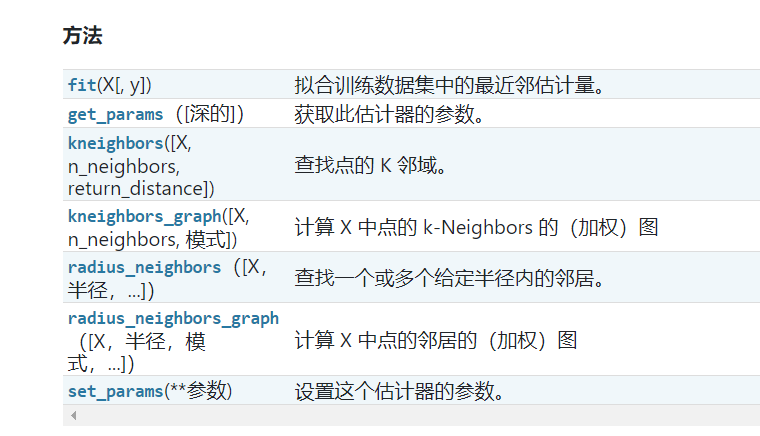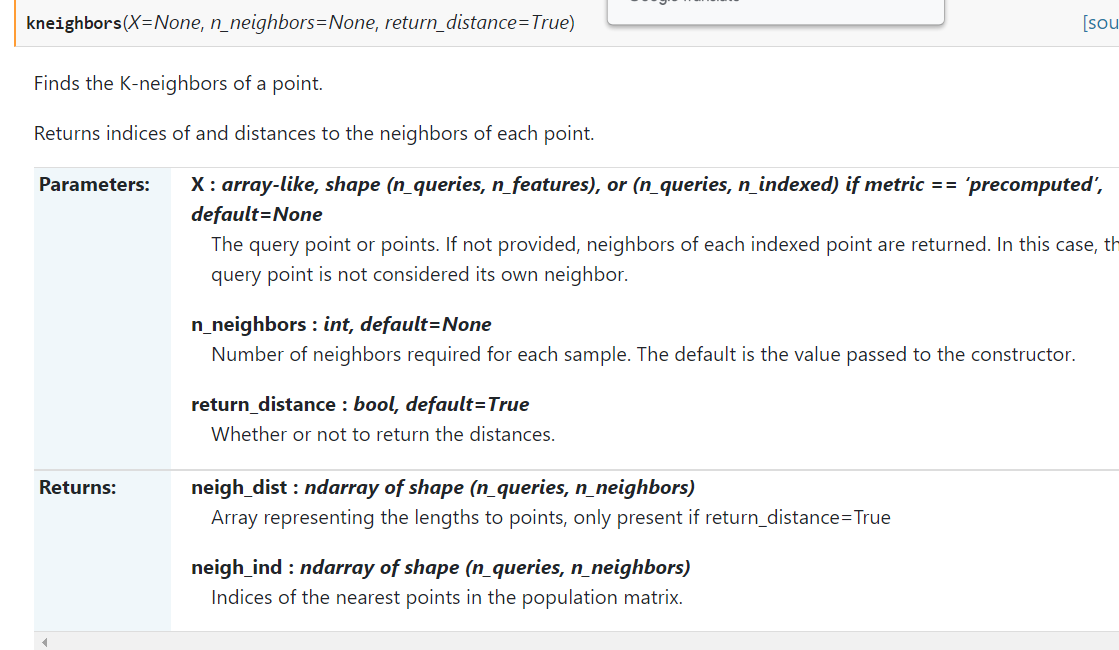• 运行结果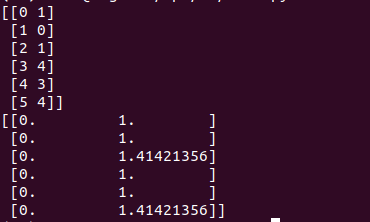• 加入一行代码，直接抽象表示每个点的2个邻居
`>>> nbrs.kneighbors_graph(X).toarray()`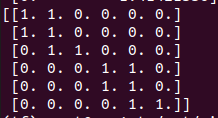## 0x02.2 KNN下的监督学习

``````from sklearn.neighbors import KNeighborsClassifier
x=[,,,]
y=[0,0,1,1]
neigh=KNeighborsClassifier(n_neighbors=3)
neigh.fit(x,y)
print(neigh.predict([[1.1]]))
print(neigh.predict_proba([[0.9]]))
``````

neigh.predict 去预测 [1.1] 会被分成什么类

• 正常运行结果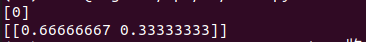• 修改了数据进行标签预测后